NEET  >  NCERT Solutions: Structure of Atom- 3

# NCERT Solutions: Structure of Atom- 3 Notes | Study Additional Documents & Tests for NEET - NEET

## Document Description: NCERT Solutions: Structure of Atom- 3 for NEET 2022 is part of Additional Documents & Tests for NEET preparation. The notes and questions for NCERT Solutions: Structure of Atom- 3 have been prepared according to the NEET exam syllabus. Information about NCERT Solutions: Structure of Atom- 3 covers topics like and NCERT Solutions: Structure of Atom- 3 Example, for NEET 2022 Exam. Find important definitions, questions, notes, meanings, examples, exercises and tests below for NCERT Solutions: Structure of Atom- 3.

Introduction of NCERT Solutions: Structure of Atom- 3 in English is available as part of our Additional Documents & Tests for NEET for NEET & NCERT Solutions: Structure of Atom- 3 in Hindi for Additional Documents & Tests for NEET course. Download more important topics related with notes, lectures and mock test series for NEET Exam by signing up for free. NEET: NCERT Solutions: Structure of Atom- 3 Notes | Study Additional Documents & Tests for NEET - NEET
 1 Crore+ students have signed up on EduRev. Have you?

Question 2.53: The ejection of the photoelectron from the silver metal in the photoelectric effect experiment can be stopped by applying the voltage of 0.35 V when the radiation 256.7 nm is used. Calculate the work function for silver metal.

Ans:- From the principle of conservation of energy, the energy of an incident photon (E) is equal to the sum of the work function (W0) of radiation and its kinetic energy (K.E) i.e.,

E = W0  K.E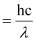⇒ W0 = E – K.E

Energy of incident photon (E)

Where,

c = velocity of radiation

h = Planck’s constant

λ = wavelength of radiation

Substituting the values in the given expression of E: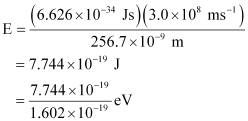E = 4.83 eV

The potential applied to silver metal changes to kinetic energy (K.E) of the photoelectron. Hence,

K.E = 0.35 V

K.E= 0.35 eV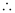Work function, W0 = E – K.E

= 4.83 eV – 0.35 eV

= 4.48 eV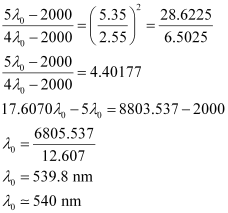Question 2.54: If the photon of the wavelength 150 pm strikes an atom and one of its inner bound electrons is ejected out with a velocity of 1.5 × 107 ms–1, calculate the energy with which it is bound to the nucleus.

Ans:- Energy of incident photon (E) is given by,

E = hc / λ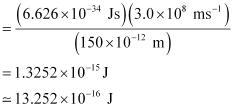Energy of the electron ejected (K.E)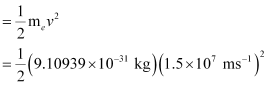= 10.2480 × 10–17 J = 1.025 × 10–16J

Hence, the energy with which the electron is bound to the nucleus can be obtained as:

E – K.E = 13.252 × 10–16 J – 1.025 × 10–16 J

= 12.227 × 10–16 J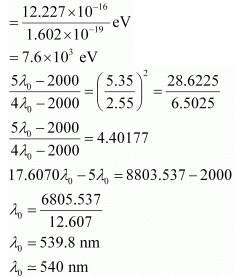Question 2.55: Emission transitions in the Paschen series end at orbit n = 3 and start from orbit n and can be represented as = 3.29 × 1015 (Hz) [1/32 – 1/n2] . Calculate the value of n if the transition is observed at 1285 nm. Find the region of the spectrum.

Ans:- Wavelength of transition = 1285 nm

= 1285 × 10–9 m (Given)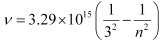(Given)

Since  v = c/λ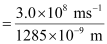ν = 2.33 × 1014 s–1

Substituting the value of ν in the given expression,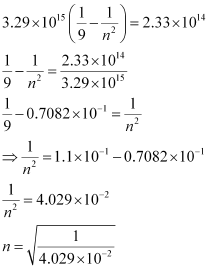n = 4.98 ≈ 5

Hence, for the transition to be observed at 1285 nm, n = 5.

The spectrum lies in the infra-red region.

Question 2.56: Calculate the wavelength for the emission transition if it starts from the orbit having radius 1.3225 nm & ends at 211.6 pm. Name the series to which this transition belongs & the region of the spectrum.

Ans:- The radius of the nth orbit of hydrogen-like particles is given by,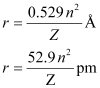For radius (r1) = 1.3225 nm

= 1.32225 × 10–9 m

= 1322.25 × 10–12 m

= 1322.25 pm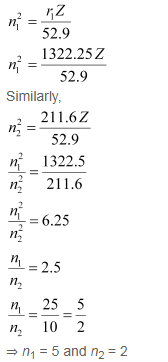Thus, the transition is from the 5th orbit to the 2nd orbit. It belongs to the Balmer series.

Wave number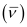for the transition is given by,

1.097 × 107 m–1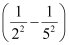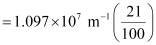= 2.303 × 106 m–1Wavelength (λ) associated with the emission transition is given by,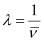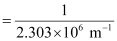= 0.434 ×10–6 m

λ = 434 nm

Question 2.57: Dual behaviour of matter proposed by de Broglie led to the discovery of electron microscope often used for the highly magnified images of biological molecules & other type of material. If the velocity of the electron in this microscope is 1.6×106 ms–1, calculate de Broglie wavelength associated with this electron.

Ans:- From de Broglie’s equation,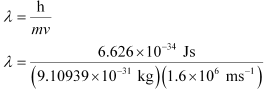= 4.55 × 10–10 m = 455 pmde Broglie’s wavelength associated with the electron is 455 pm.

Question 2.58: Similar to electron diffraction, neutron diffraction microscope is also used for the determination of the structure of molecules. If the wavelength used here is 800 pm, calculate the characteristic velocity associated with the neutron.

Ans:- From de Broglie’s equation,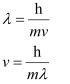Where,

v = velocity of particle (neutron)

h = Planck’s constant

m = mass of particle (neutron)

λ = wavelength

Substituting the values in the expression of velocity (v),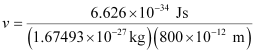= 4.94 × 102 ms–1

v = 494 ms–1Velocity associated with the neutron = 494 ms–1

Question 2.59: If the velocity of the electron in Bohr’s first orbit is 2.19 × 106 ms–1, calculate the de Broglie wavelength associated with it.

Ans:- According to de Broglie’s equation,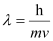Where,

λ = wavelength associated with the electron

h = Planck’s constant

m = mass of electron

v = velocity of electron

Substituting the values in the expression of λ: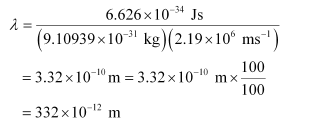λ = 332 pmWavelength associated with the electron = 332 pm

Question 2.60: The velocity associated with a proton moving in a potential difference of 1000 V is 4.37 × 105 ms–1. If the hockey ball of mass 0.1 kg is moving with this velocity, calculate the wavelength associated with this velocity.

Ans:- According to De Broglie’s expression,

λ = h/mv

Substituting the values in the expression,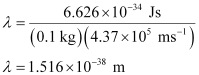Question 2.61: If the position of the electron is measured within an accuracy of 0.002 nm, calculate the uncertainty in the momentum of the electron. Suppose the momentum of the electron is h/4πm × 0.05 nm, is there any problem in defining this value.

Ans:- From Heisenberg’s uncertainty principle,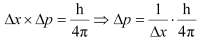Where,

Δx = uncertainty in position of the electron

Δp = uncertainty in momentum of the electron

Substituting the values in the expression of Δp: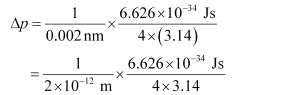= 2.637 × 10–23 Jsm–1

Δp = 2.637 × 10–23 kgms–1 (1 J = 1 kgms2s–1)Uncertainty in the momentum of the electron = 2.637 × 10–23 kgms–1.

Actual momentum =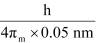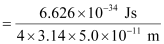= 1.055 × 10–24 kgms–1

Since the magnitude of the actual momentum is smaller than the uncertainty, the value cannot be defined.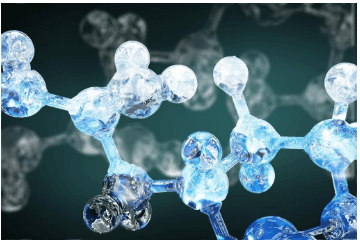Question 2.62: The quantum numbers of six electrons are given below. Arrange them in order of increasing energies. If any of these combination(s) has/have the same energy lists:

(1). n = 4, l = 2, ml = –2 , ms = –1/2

(2). n = 3, l = 2, ml= 1 , ms = 1/2

(3). n = 4, l = 1, ml = 0 , ms = 1/2

(4). n = 3, l = 2, ml = –2 , ms = –1/2

(5). n = 3, l = 1, ml = –1 , ms= 1/2

(6). n = 4, l = 1, ml = 0 , ms = 1/2

Ans:- For = 4 and l = 2, the orbital occupied is 4d.

For = 3 and l = 2, the orbital occupied is 3d.

For = 4 and l = 1, the orbital occupied is 4p.

Hence, the six electrons i.e., 1, 2, 3, 4, 5, and 6 are present in the 4d, 3d, 4p, 3d, 3p, and 4p orbitals respectively.

Therefore, the increasing order of energies is 5(3p) < 2(3d) = 4(3d) < 3(4p) = 6(4p) < 1 (4d).

Question 2.63: The bromine atom possesses 35 electrons.It contains 6 electrons in 2p orbital,6 electrons in 3p orbital & 5 electrons in 4p orbital. Which of these electron experiences the lowest effective nuclear charge?

Ans:- Nuclear charge experienced by an electron (present in a multi-electron atom) is dependant upon the distance between the nucleus and the orbital, in which the electron is present. As the distance increases, the effective nuclear charge also decreases.

Among p-orbitals, 4p orbitals are farthest from the nucleus of bromine atom with ( 35) charge. Hence, the electrons in the 4p orbital will experience the lowest effective nuclear charge. These electrons are shielded by electrons present in the 2p and 3p orbitals along with the s-orbitals. Therefore, they will experience the lowest nuclear charge.

Question 2.64: Among the following pairs of orbitals which orbital will experience the larger effective nuclear charge?

(i) 2s and 3s,
(ii) 4and 4f,
(iii) 3d and 3p

Ans:- Nuclear charge is defined as the net positive charge experienced by an electron in the orbital of a multi-electron atom. The closer the orbital, the greater is the nuclear charge experienced by the electron (s) in it.

(i) The electron(s) present in the 2s orbital will experience greater nuclear charge (being closer to the nucleus) than the electron(s) in the 3s orbital.

(ii) 4d will experience greater nuclear charge than 4f since 4d is closer to the nucleus.

(iii) 3p will experience greater nuclear charge since it is closer to the nucleus than 3f.

Question 2.65: The unpaired electrons in Al and Si are present in 3p orbital. Which electrons will experience more effective nuclear charge from the nucleus?

Ans:- Nuclear charge is defined as the net positive charge experienced by an electron in a multi-electron atom.The higher the atomic number,the higher is the nuclear charge. Silicon has 14 protons while aluminium has 13 protons. Hence, silicon has a larger nuclear charge of ( 14) than aluminium, which has a nuclear charge of ( 13) Thus, the electrons in the 3p orbital of silicon will experience a more effective nuclear charge than aluminium.

Question 2.66: Indicate the number of unpaired electrons in:

(a) P,

(b) Si,

(c) Cr,

(d) Fe

(e) Kr.

Ans:- (a) Phosphorus (P):

Atomic number = 15

The electronic configuration of P is:

1s2 2s2 2p6 3s2 3p3

The orbital picture of P can be represented as: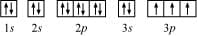From the orbital picture, phosphorus has three unpaired electrons.

(b) Silicon (Si):

Atomic number = 14

The electronic configuration of Si is:

1s2 2s2 2p6 3s2 3p2

The orbital picture of Si can be represented as: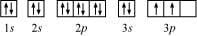From the orbital picture, silicon has two unpaired electrons.

(c) Chromium (Cr):

Atomic number = 24

The electronic configuration of Cr is:

1s2 2s2 2p6 3s2 3p4s1 3d5

The orbital picture of chromium is: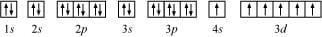From the orbital picture, chromium has six unpaired electrons.

(d) Iron (Fe):

Atomic number = 26

The electronic configuration is:

1s2 2s2 2p6 3s2 3p4s3d6

The orbital picture of chromium is: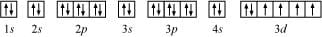From the orbital picture, iron has four unpaired electrons.

(e) Krypton (Kr):

Atomic number = 36

The electronic configuration is:

1s2 2s2 2p6 3s2 3p4s3d10 4p6

The orbital picture of krypton is: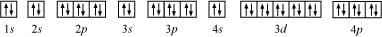Since all orbitals are fully occupied, there are no unpaired electrons in krypton.

Question 2.67: (a) How many sub-shells are associated with = 4?

(b) How many electrons will be present in the sub-shells having ms value of –1/2 for n = 4?

Ans:- (a) n = 4 (Given)

For a given value of ‘n’, ‘l’ can have values from zero to (n – 1).

= 0, 1, 2, 3

Thus, four sub-shells are associated with n = 4, which are s, p, d and f.

(b) Number of orbitals in the nth shell = n2

For n = 4

Number of orbitals = 16

If each orbital is taken fully, then it will have 1 electron with ms value of - ½.

∴ Number of electrons with ms value of  (- ½ ) is 16.

The document NCERT Solutions: Structure of Atom- 3 Notes | Study Additional Documents & Tests for NEET - NEET is a part of the NEET Course Additional Documents & Tests for NEET.
All you need of NEET at this link: NEET

## Additional Documents & Tests for NEET

10 videos|153 docs|55 tests
 Use Code STAYHOME200 and get INR 200 additional OFF

## Additional Documents & Tests for NEET

10 videos|153 docs|55 tests

### How to Prepare for NEET

Read our guide to prepare for NEET which is created by Toppers & the best Teachers

Track your progress, build streaks, highlight & save important lessons and more!

,

,

,

,

,

,

,

,

,

,

,

,

,

,

,

,

,

,

,

,

,

;